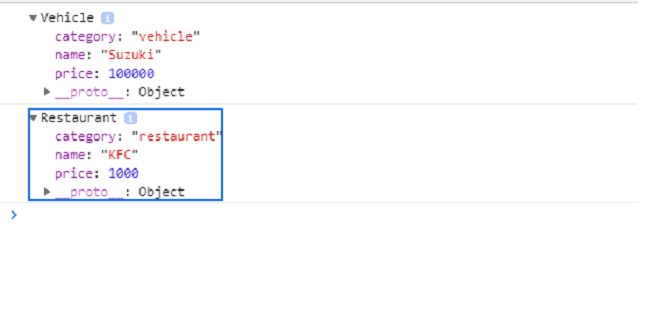Related Articles
JavaScript Function.prototype.call() Method
• Last Updated : 31 Aug, 2020

The call() method allows function calls belonging to one object to be assigned and it is called for a different object. It provides a new value of this to the function. The call() method allows you to write a method once and allows it for inheritance in another object, without rewriting the method for the new object.

Syntax:

`myFunc.call([thisArg[, arg1, arg2, ...argN]])`

Parameters:

• thisArg: The values to use as this when calling myFunc function.
• arg1, arg2, …, argN: These are the arguments for the above mentioned function.

Note: In certain cases, thisArg may not be the actual value. If the method is in non-strict mode, primitive values will be converted to objects and null,undefined will be replaced with the global object.

Return value: It returns the specified this value and arguments as a result of calling of the function.

Example 1: The following example demonstrates the use of calling to chain constructors for an object.

 `<``script``>``function Product(name,``     ``price) {``    ``this.name = name;``    ``this.price = price;``  ``}``   ` `  ``function Vehicle(name, ``           ``price) {``   ``Product.call(this,``           ``name, price);``    ``this.category = 'vehicle';``  ``}``   ` `  ``function Restaurant(name,``           ``price) {``   ``Product.call(this, ``           ``name, price);``    ``this.category = 'restaurant';``  ``}``   ` `  ``const car = new Vehicle('Suzuki',``        ``100000);``  ``const restau = new ``        ``Restaurant('KFC', 1000);`` ` `  ``console.log(car);``  ``console.log(restaurant);```

Output:Example 2: The following example demonstrates the use of call() method to invoke an anonymous function.

 `<``script``>``const Birds = [``    ``{ species: 'Peagion', name: 'King' },``    ``{ species: 'Crow', name: 'Fail' }``  ``];``   ` `let i=0;`` ``while(i<``Birds.length``){ ``  ``(function(i) {``    ``this.print` `= ``function``() {``     ``console.log('#' + i + ' ' ``            ``+ this.species``              ``+ ': ' + this.name);``      ``}``      ``this.print();``    ``}).call(Birds[i], i);``    ``++i;``  ``}```

Output:

```#0 Peagion: King
#1 Crow: Fail
```

Example 3: The following example demonstrates the use of call method to invoke a function and specifying the context for ‘this’.

 `<``script``>``function greet() {``  ``const reply = [this.animal, ``    ``'typically sleep between',``        ``this.sleepDuration].join(' ');``    ``console.log(reply);``  ``}``   ` `  ``const obj = {``    ``animal: 'Rats', ``    ``sleepDuration: '2 and 5 hours'``  ``};``   ` `  ``greet.call(obj);```

Output:

``` Rats typically sleep between 2 and 5 hours
```

Example 4: The following example demonstrates the use of call() method to invoke a function without specifying the first argument.

 `<``script``>``var str = 'Brad';`` ` `function display() {``  ``console.log('string value is %s ',``     ``this.str);``}`` ` `display.call();```

Output:

``` string value is Brad
```

Note: In strict mode, the value of this will be undefined.

 `<``script``>``'use strict';`` ` `var str = 'Brad';`` ` `function display() {``  ``console.log('str value is %s ', ``   ``this.str);``}``display.call();```

Output:

```Cannot read property 'str' of undefined
```
My Personal Notes arrow_drop_up